### Home > CALC > Chapter 8 > Lesson 8.1.3 > Problem8-32

8-32.
1.No calculator! Integrate. Homework Help ✎

1.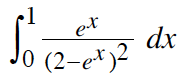2.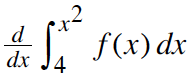3.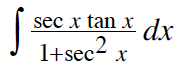4.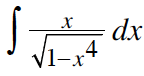5.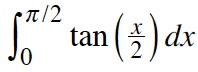2.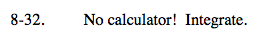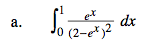Let U = 2 − ex.

Don't forget to rewrite the bounds in terms of u.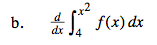The derivative of an INDEFINITE integral, is the original function (FTC, pt.1) But the derivative of a DEFINITE integral is the original function times the derivative of the bounds.

2xf(x2)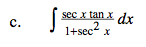Let u = sec(x).

arctan(u) + C =
Now rewrite the answer in terms of x.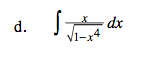Recall that x4 = (x2)2.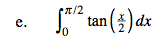$\int_{0}^{\frac{\pi }{2}}\frac{\text{sin}\frac{x}{2}}{\text{cos}\frac{x}{2}}dx=$

Use u-substitution.
And don't forget about the bounds!